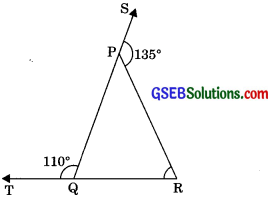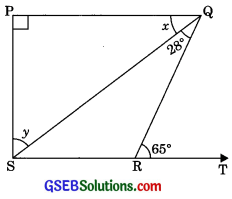# GSEB Solutions Class 9 Maths Chapter 6 Lines and Angles Ex 6.3

Gujarat Board GSEB Solutions Class 9 Maths Chapter 6 Lines and Angles Ex 6.3 Textbook Questions and Answers.

## Gujarat Board Textbook Solutions Class 9 Maths Chapter 6 Lines and Angles Ex 6.3

Question 1.
In figure sides QP and RQ of ΔPQR are produced to points S and T respectively. If ∠SPR = 135° and ∠PQT = 110°, find ∠PRQ.Solution:
∠PQT + ∠PQR = 180° (Linear pair)
⇒ 110° + ∠PQR = 180°
⇒ ∠PQR = 180° – 110°
⇒ ∠PQR = 70°
∠RPS = ∠PQR + ∠PRQ (Exterior angle property)
⇒ 135° – 70° + ∠PRQ
⇒ ∠PRQ = 135° – 70°
∠PRQ = 65°Question 2.
In figure ∠X = 62°, ∠XYZ = 54°. If YO and ZO are the bisectors of ∠XYZ and ∠XZY respectively of ΔXYZ, find ∠OZY and ∠YOZ.Solution:
In ΔXYZ,
∠YXZ + ∠XYZ + ∠XZY = 180° (Angle sum property)
⇒ 62° + 54° + ∠XZY = 180°
⇒ 116° + ∠XZY = 180°
⇒ ∠XZY = 180° – 116°
∠XZY = 64°
∠OZY = $$\frac {∠XZY}{2}$$ = $$\frac {64°}{2}$$ (OZ is bisector of ∠XZY)
∠OYZ = 32°
∠OZY = $$\frac {∠XZY}{2}$$
(OY is bisector of ∠XYZ)
∠OYZ = $$\frac {54°}{2}$$
∠OYZ = 27°

In ΔOYZ,
∠OYZ + ∠OZY + ∠YOZ = 180°
(Angle sum property)
⇒ 27° + 32° + ∠YOZ = 180°
⇒ 59° + ∠YOZ = 180°
⇒ ∠YOZ = 180° – 59°
⇒ ∠YOZ = 121°Question 3.
In figure AB || DE, ∠BAC = 35° and ∠CDE = 53°, find ∠DCE.
Solution:
AB || DE
∠DEC = ∠BAC
(Alternate interior angles)
⇒ ∠DEC = 35°Now in ACDE,
∠DCE + ∠CDE + ∠DEC = 180° (Angle sum property)
∠DCE + 53° + 35° = 180°
∠DCE = 180° – 88° ∠DCE = 92°

Question 4.
In figure, if lines PQ and RS intersect at point T, such that ∠PRT = 40°, ∠RPT = 95° and ∠TSQ = 75°, find ∠SQT.
Solution:
In ΔPRT,
∠PRT + ∠RPT + ∠PTR = 180° (Angle sum property)
40° + 95° + ∠PTR = 180°
135° + ∠PTR = 180°
PTR = 180° – 135° ∠PTR = 45°
∠STQ = ∠PTR
(Vertically opposite angles)
∠STQ = 45°Now in ΔSQT,
∠STQ + ∠SQT ∠QST = 180° (Angle sum property)
⇒ 45° + ∠SQT + 75° = 180°
⇒ ∠SQT + 120° – 180°
⇒ ∠SQT = 180° – 120°
⇒ ∠SQT = 60°Question 5.
In figure, if PQ ⊥PS, PQ || SR, ∠SQR = 28° and ∠QRT = 65°, then find the values of x and y.Solution:
We have PQ || SR
∴ PQ || ST
∴ ∠PQR = ∠QTR (Alternate interior angles)
⇒ x + 28° = 65°
⇒ x = 65° – 28°
⇒ x = 37°

In ΔPQS,
∠PQS + ∠PSQ + ∠SPQ = 180° (Angle sum property)
⇒ x + y + 90° = 180°
37° + 90° + y = 180°
127° + y = 180°
⇒ y= 180° – 127°
⇒ y = 53°Question 6.
In figure, the side QR of APQR is produced to a point S. If the bisectors of ∠PQR and ∠PRS meet at point T, then prove that ∠QTR = $$\frac {1}{2}$$ ∠QPR.Solution:
Given: ΔPQR in which side QR produced to S and bisectors of ∠QPRPQR and ∠QPRPRS meet at T.
To Prove: ∠QPR = $$\frac {1}{2}$$ ∠QPR
Proof: ∠TRS is the exterior angle of ΔTQR.
∴ ∠TRS = ∠1 + ∠2 …….(1)
(Exterior angle of triangle is equal to sum of two interior opposite angles)
∠PRS is an exterior angle of ΔPQR.
∴ ∠PRS = ∠PQR + ∠QPR
(Exterior angle of a triangle is equal to the sum of its two interior opposite angles)
⇒ 2 ∠TRS = 2∠1 + ∠QPR
2(∠1 + ∠2) = 2∠1 + ∠QPR [From eqn(Δ)]
⇒ 2∠1 + 2∠2 = 2∠1 + ∠QPR
⇒ 2∠2 = ∠QPR
⇒ ∠2 = $$\frac {1}{2}$$ ∠QPR
⇒ ∠QTR = $$\frac {1}{2}$$ ∠QPR